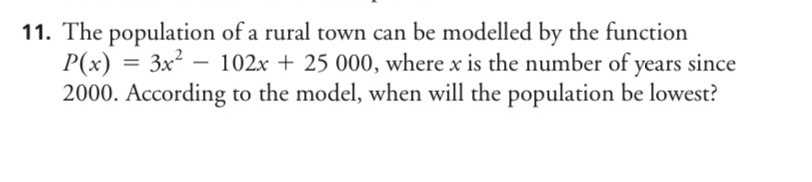### ¿Todavía tienes preguntas de matemáticas?

Pregunte a nuestros tutores expertos
Algebra
Pregunta11. The population of a rural town can be modelled by the function$$P ( x ) = 3 x ^ { 2 } - 102 x + 25000 ,$$ where $$x$$ is the number of years since $$2000$$ . According to the model, when will the population be lowest?